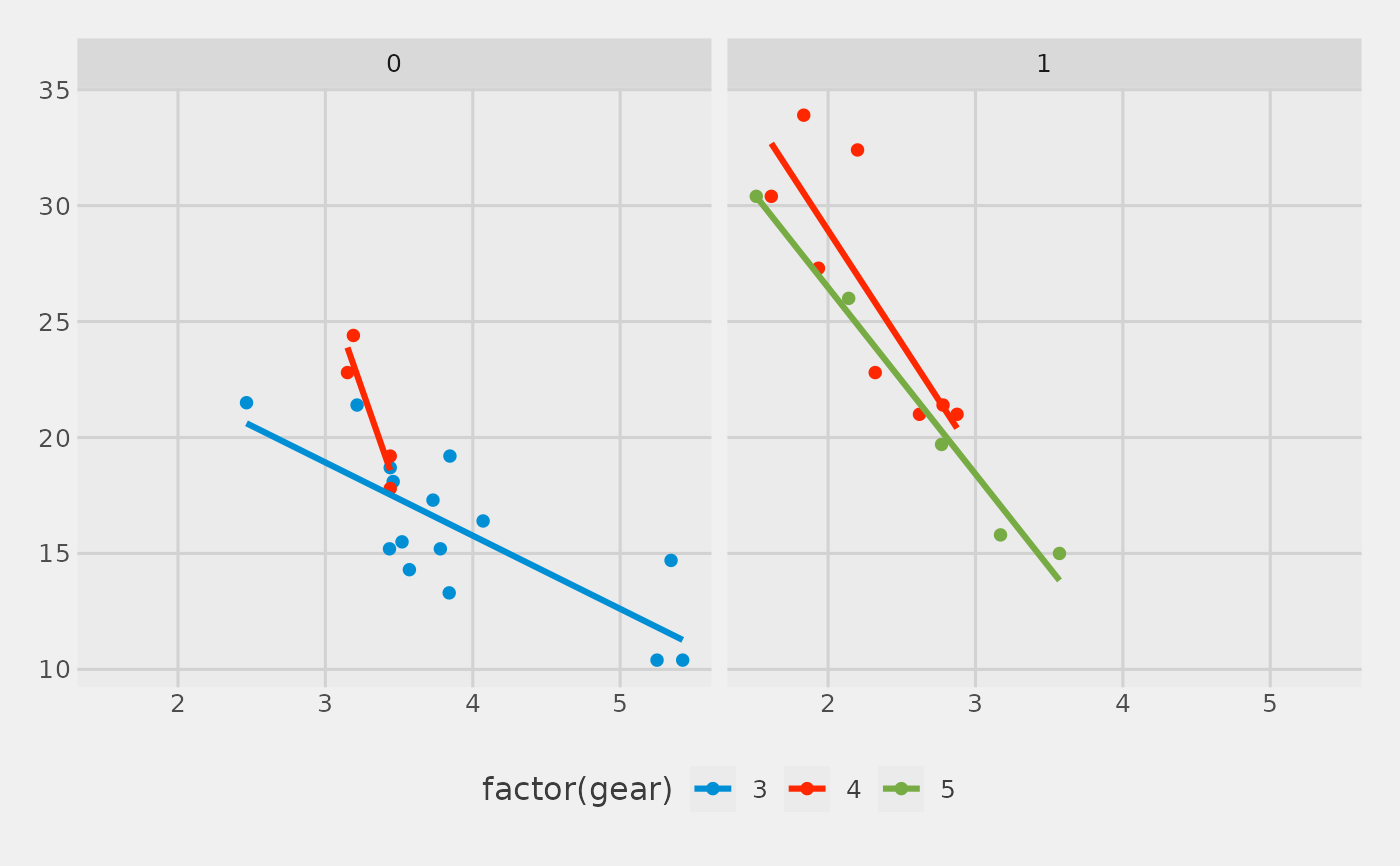Theme inspired by the plots on FiveThirtyEight.

theme_fivethirtyeight(base_size = 12, base_family = "sans")

## Arguments

base_size base font size, given in pts. base font family

## Examples

library("ggplot2")
p <- ggplot(mtcars, aes(x = wt, y = mpg, colour = factor(gear))) +
geom_point() +
facet_wrap(~am) +
geom_smooth(method = "lm", se = FALSE) +
scale_color_fivethirtyeight() +
theme_fivethirtyeight()
p
#> geom_smooth() using formula 'y ~ x'